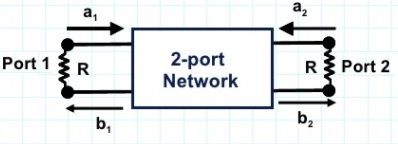# Scattering Matrix in Microwave Engineering

### Microwave Hybrid Circuits:

A microwave network or microwave hybrid circuit consist of several microwave devices such as sources, attenuators, filters, amplifiers etc coupled together by transmission lines for the transmission of microwave signal. The point of interconnection of two or more devices is known as a junction. The measurement of z,y,h and ABCD parameter is difficult at microwave frequencies due to following reasons.

1. Non availability of voltage and current measuring equipments.
2. Short circuit not easily achieved for wide range of frequencies.
3. Presence of active devices make the circuit unstable so microwave circuits are analyzed using scattering parameters or ‘S’ matrix. S matrix relates the amplitude of reflected waves with incident waves.

### Scattering Matrix in Microwave Engineering :

It is a square matrix which gives all the combinations of power relationship between input and output ports of a microwave junction. The elements of ‘S’ matrix are known as scattering parameters or scattering coefficients.Microwave 2 port network
Consider the microwave 2 port network.

a1 – amplitude of incident wave at port 1
a2 – amplitude of incident wave at port 2
b1 – amplitude of reflected wave at port 1
b2 – amplitude of reflected wave at port 2

The incident and reflected waves can be related using ‘S‘ matrix as
[b] = [s] [a]

b1 = s11a1 + s12a2
b2 = s21a1 + s22a2

s11 is the reflection coefficient at port 1 when, a2 = 0
s11 = b1/a1 where a2 = 0

s22 is the reflection coefficient at port 2 when, a1 = 0
s22 = b2/a2 where a1 = 0

s12 is the attenuation of wave travelling from port 2 to port 1 when, a1 = 0
s12 = b1/a2 where a1 = 0

s21 is the attenuation of wave travelling from port 1 to port 2 when, a2 = 0
s21 = b2/a1 where a2 = 0

In a microwave network if the incident power is Pi, reflected power is Pr, output power is Pa then, the losses defined are

1. Insertion loss = 10 log (Pi/Po)
2. Transmission loss or attenuation = 10 log((Pi - Pr)/Po)
3. Reflection loss = 10 log (Pi/(Pi - Pr))
4. Return loss = 10 log (Pi/Pr)

### Properties of S matrix:

1. S matrix is always a square matrix of order n x n.

2. Under perfect match condition the diagonal elements of S matrix are zero.

3. S matrix is always symmetric. ie, Sij = Sji

4. S matrix is an unitary matrix. Ie, [S][S]*= I. where, I is an identity matrix.

5. The sum of product of each term of any row or column multiplied by complex conjugate of corresponding term of another row or column is zero.

6. In a two port network, if the reference plane are shifted from one and two to 1’ and 2’ the new S matrix is given by

This property is known as phase shift property.

7. Since S matrix is symmetric [S]T = [S]

Sreejith Hrishikesan

Sreejith Hrishikesan is a ME post graduate and has been worked as an Assistant Professor in Electronics Department in KMP College of Engineering, Ernakulam. For Assignments and Projects, Whatsapp on 8289838099.